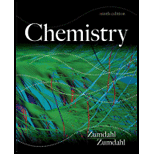# Consider the system A ( g ) → B ( g ) at25°C. a. Assuming that G A o = 8996 J/mol and G B o = 11 , 718 J/mol , calculate the value of the equilibrium constant for this reaction. b. Calculate the equilibrium pressures that result if 1.00 mole of A( g ) at 1.00 atm and 1.00 mole of B( g ) at 1.00 atm are mixed at 25°C. c. Show by calculations that ∆G = 0 at equilibrium.### Chemistry

9th Edition
Steven S. Zumdahl
Publisher: Cengage Learning
ISBN: 9781133611097### Chemistry

9th Edition
Steven S. Zumdahl
Publisher: Cengage Learning
ISBN: 9781133611097

#### Solutions

Chapter
Section
Chapter 17, Problem 109CP
Textbook Problem

## Expert Solution

### Want to see the full answer?

Check out a sample textbook solution.See solution

### Want to see this answer and more?

Experts are waiting 24/7 to provide step-by-step solutions in as fast as 30 minutes!*

See Solution

*Response times vary by subject and question complexity. Median response time is 34 minutes and may be longer for new subjects.